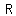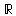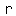# U+211D DOUBLE-STRUCK CAPITAL R

U+211D was added to Unicode in version 1.1 (1993). It belongs to the blockLetterlike Symbols in the Basic Multilingual Plane.

This character is a Uppercase Letter and is commonly used, that is, in no specific script. The character is also known as the set of real numbers.

The glyph is a Font composition of the glyphs. It has a Neutral East Asian Width. In bidirectional context it acts as Left To Right and is not mirrored. The glyph can, under circumstances, be confused with 24 other glyphs. In text U+211D behaves as Alphabetic regarding line breaks. It has type Upper for sentence and ALetter for word breaks. The Grapheme Cluster Break is Any.

In mathematics, a real number is a value that represents a quantity along a continuous line. The adjective real in this context was introduced in the 17th century by Descartes, who distinguished between real and imaginary roots of polynomials.

The real numbers include all the rational numbers, such as the integer −5 and the fraction 4/3, and all the irrational numbers such as √2 (1.41421356…, the square root of two, an irrational algebraic number) and π (3.14159265…, a transcendental number). Real numbers can be thought of as points on an infinitely long line called the number line or real line, where the points corresponding to integers are equally spaced. Any real number can be determined by a possibly infinite decimal representation such as that of 8.632, where each consecutive digit is measured in units one tenth the size of the previous one. The real line can be thought of as a part of the complex plane, and complex numbers include real numbers.

These descriptions of the real numbers are not sufficiently rigorous by the modern standards of pure mathematics. The discovery of a suitably rigorous definition of the real numbers – indeed, the realization that a better definition was needed – was one of the most important developments of 19th century mathematics. The currently standard axiomatic definition is that real numbers form the unique Archimedean complete totally ordered field (R ; + ; · ; <), up to an isomorphism, whereas popular constructive definitions of real numbers include declaring them as equivalence classes of Cauchy sequences of rational numbers, Dedekind cuts, or certain infinite "decimal representations", together with precise interpretations for the arithmetic operations and the order relation. These definitions are equivalent in the realm of classical mathematics.

The reals are uncountable; that is: while both the set of all natural numbers and the set of all real numbers are infinite sets, there can be no one-to-one function from the real numbers to the natural numbers: the cardinality of the set of all real numbers (denoted and called cardinality of the continuum) is strictly greater than the cardinality of the set of all natural numbers (denoted ). The statement that there is no subset of the reals with cardinality strictly greater than and strictly smaller than is known as the continuum hypothesis (CH). It is known to be neither provable nor refutable using the axioms of Zermelo–Fraenkel set theory (ZF), the standard foundation of modern mathematics, in the sense that some models of ZF satisfy CH while others violate it.

## Representations

System Representation
8477
UTF-8 E2 84 9D
UTF-16 21 1D
UTF-32 00 00 21 1D
URL-Quoted %E2%84%9D
HTML-Escape &#x211D;
Wrong windows-1252 Mojibake â
HTML-Escape &reals;
HTML-Escape &Ropf;
alias the set of real numbers
LaTeX \mathbb{R}

## Complete Record

Property Value
Age (age) 1.1
Unicode Name (na) DOUBLE-STRUCK CAPITAL R
Unicode 1 Name (na1) DOUBLE-STRUCK R
Block (blk) Letterlike_Symbols
General Category (gc) Uppercase Letter
Script (sc) Common
Bidirectional Category (bc) Left To Right
Combining Class (ccc) Not Reordered
Decomposition Type (dt) Font
Decomposition Mapping (dm)Lowercase (Lower)
Simple Lowercase Mapping (slc)Lowercase Mapping (lc)Uppercase (Upper)
Simple Uppercase Mapping (suc)Uppercase Mapping (uc)Simple Titlecase Mapping (stc)Titlecase Mapping (tc)Case Folding (cf)ASCII Hex Digit (AHex)
Alphabetic (Alpha)
Bidi Control (Bidi_C)
Bidi Mirrored (Bidi_M)
Bidi Paired Bracket (bpb)Bidi Paired Bracket Type (bpt) None
Cased (Cased)
Composition Exclusion (CE)
Case Ignorable (CI)
Full Composition Exclusion (Comp_Ex)
Changes When Casefolded (CWCF)
Changes When Casemapped (CWCM)
Changes When NFKC Casefolded (CWKCF)
Changes When Lowercased (CWL)
Changes When Titlecased (CWT)
Changes When Uppercased (CWU)
Dash (Dash)
Deprecated (Dep)
Default Ignorable Code Point (DI)
Diacritic (Dia)
East Asian Width (ea) Neutral
Extender (Ext)
FC NFKC Closure (FC_NFKC)Grapheme Cluster Break (GCB) Any
Grapheme Base (Gr_Base)
Grapheme Extend (Gr_Ext)
Hex Digit (Hex)
Hangul Syllable Type (hst) Not Applicable
Hyphen (Hyphen)
ID Continue (IDC)
Ideographic (Ideo)
ID Start (IDS)
IDS Binary Operator (IDSB)
IDS Trinary Operator and (IDST)
InMC (InMC)
Indic Positional Category (InPC) NA
Indic Syllabic Category (InSC) Other
ISO 10646 Comment (isc)
Joining Group (jg) No_Joining_Group
Join Control (Join_C)
Jamo Short Name (JSN)
Joining Type (jt) Non Joining
Line Break (lb) Alphabetic
Logical Order Exception (LOE)
Math (Math)
Noncharacter Code Point (NChar)
NFC Quick Check (NFC_QC) Yes
NFD Quick Check (NFD_QC) Yes
NFKC Casefold (NFKC_CF)NFKC Quick Check (NFKC_QC) No
NFKD Quick Check (NFKD_QC) No
Numeric Type (nt) None
Numeric Value (nv) NaN
Other Alphabetic (OAlpha)
Other Default Ignorable Code Point (ODI)
Other Grapheme Extend (OGr_Ext)
Other ID Continue (OIDC)
Other ID Start (OIDS)
Other Lowercase (OLower)
Other Math (OMath)
Other Uppercase (OUpper)
Pattern Syntax (Pat_Syn)
Pattern White Space (Pat_WS)
Quotation Mark (QMark)
Sentence Break (SB) Upper
Simple Case Folding (scf)Script Extension (scx) Common
Soft Dotted (SD)
STerm (STerm)
Terminal Punctuation (Term)
Unified Ideograph (UIdeo)
Variation Selector (VS)
Word Break (WB) ALetter
White Space (WSpace)
XID Continue (XIDC)
XID Start (XIDS)
Expands On NFC (XO_NFC)
Expands On NFD (XO_NFD)
Expands On NFKC (XO_NFKC)
Expands On NFKD (XO_NFKD)